# Benz plane

(diff) ← Older revision | Latest revision (diff) | Newer revision → (diff)
Jump to: navigation, search

circle plane

A common concept for the geometries of Möbius, Laguerre and Lie, and Minkowski, as given by W. Benz in [a1].

A Benz plane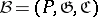consist of a set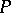of points and two sets of subsets of, namely the setof generators and the set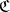of circles, satisfying:

1) each generator intersects each circle in exactly one point;

2) through any three points no two of which are on a common generator there passes exactly one circle;

3) ifis a circle,is a point on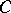and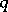is a point outsideand not on a common generator with, then there is exactly one circle throughintersectingatonly (the tangency axiom);

4) there is a circle, andcontains at least three points;

5a)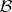is a Möbius plane if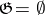;

5b)is a Laguerre plane ifis a partition of;

5c)is a Minkowski plane if, with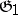and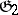partitions of, and any generator ofintersects any generator ofin exactly one point.

For a point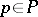one defines its derived affine plane,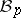, as follows. The points ofare the points different fromand outside all generators through. The lines ofare the generators not passing throughand the circles throughminus the pointitself.

So, a Benz planeis a special kind of chain space: Two (different) points ofare distant if and only if they do not belong to a common generator.

An affine model ofis obtained by adding tothe traces of all circles not through.

An imbeddable (or egg-like) Benz plane is isomorphic to a Benz plane of the formwitha subset of a (projective or affine) three-dimensional space andconsist of planar sections of:

a)is an ovoid;

b)is a cylindrical surface (cylinder) over an oval as directrix, and henceis covered by its set of generators;

c)is a ruled quadric in projective-space (a hyperbolic quadric), and hence has two sets of (mutually skew) straight lines covering it. In any case,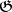consists of the generators onandconsists of the intersections ofwith all planes containing more than one point ofbut no line of. Stereographic projection once more gives an affine model of.

A classical Benz plane is a chain geometry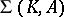where the-algebrais a-dimensional-vector space. There are three such algebras.

i)is a quadratic field extension (cf. Extension of a field). Thenis a Möbius plane.

ii)with. Thenis a Laguerre plane.

iii)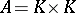(addition and multiplication componentwise). Thenis a Minkowski plane. The classical Benz planes are imbeddable. Here,becomes an elliptic quadric, a quadric cone without its vertex or a hyperbolic quadric, respectively.

The classical affine Benz planes (affine models) are as follows: There is an affine planeoverand inone takes:

I) all straight lines and circles;

II) all straight lines not belonging to a given parallel class and all parabolas having axis in this parallel class;

III) all straight lines not belonging to two given parallel classes and all hyperbolas having asymptotes in these parallel classes. Many theorems from Euclidean geometry valid in I) (e.g., the theorem on inscribed angles) also hold in II) and III), see [a4], §3.4.

A Benz plane is called Miquelian if the so-called Miquels condition is valid in it: Let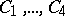be circles no three of which have a common point but such that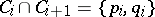for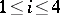(subscripts modulo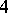), whereand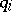are not necessarily different points. Iflie on a common circle, then so do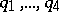.

The following theorem was proved by B.L. van der Waerden and L.J. Smid in 1935 for I), II) and by G. Kaerlain in 1970 for III): A Benz plane is classical if and only if it is Miquelian.

T.J. Kahn (1980) has proved that a Benz plane is imbeddable if and only if it satisfies the so-called bundle condition: Let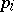,,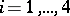, be eight points no two of which are on a common generator. If,, belong to a common circle in five cases, then this is also true for the sixth case.

A finite Benz plane is a Benz plane with a finite point set. A finite Benz planehas order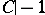for any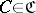. This number is independent of the choice of. The following theorem was proved by P. Dembowski (1964) for I) and by W. Heise (1974) for III): A finite Möbius or Minkowski plane of even order is imbeddable.

A direct consequence of the Segre–Barlotti theorem (that an ovoid or oval in a finite Desarguesian projective space is a quadric or conic) is that a finite imbeddable Benz plane of odd order is Miquelian.

For further information see the surveys [a2], [a5] (e.g., classification of Benz planes by automorphism groups); for topological Benz planes see [a5]. A more general concept, using unitals instead of quadrics (i.e., self-conjugate points of a Hermitian form instead of a quadratic form), arose in [a1]; for subsequent developments, see [a2]. For projectivities in Benz planes see [a3].